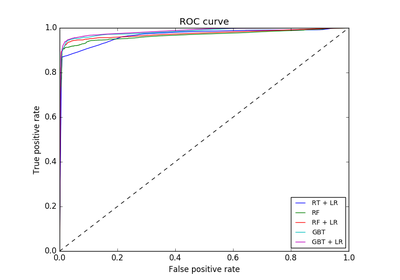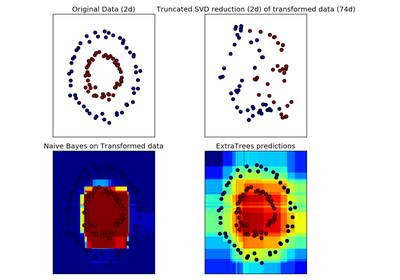# `sklearn.ensemble`.RandomTreesEmbedding¶

class `sklearn.ensemble.``RandomTreesEmbedding`(n_estimators=10, max_depth=5, min_samples_split=2, min_samples_leaf=1, min_weight_fraction_leaf=0.0, max_leaf_nodes=None, min_impurity_split=1e-07, sparse_output=True, n_jobs=1, random_state=None, verbose=0, warm_start=False)[source]

An ensemble of totally random trees.

An unsupervised transformation of a dataset to a high-dimensional sparse representation. A datapoint is coded according to which leaf of each tree it is sorted into. Using a one-hot encoding of the leaves, this leads to a binary coding with as many ones as there are trees in the forest.

The dimensionality of the resulting representation is `n_out <= n_estimators * max_leaf_nodes`. If `max_leaf_nodes == None`, the number of leaf nodes is at most `n_estimators * 2 ** max_depth`.

Read more in the User Guide.

Parameters: n_estimators : integer, optional (default=10) Number of trees in the forest. max_depth : integer, optional (default=5) The maximum depth of each tree. If None, then nodes are expanded until all leaves are pure or until all leaves contain less than min_samples_split samples. min_samples_split : int, float, optional (default=2) The minimum number of samples required to split an internal node: If int, then consider min_samples_split as the minimum number. If float, then min_samples_split is a percentage and ceil(min_samples_split * n_samples) is the minimum number of samples for each split. Changed in version 0.18: Added float values for percentages. min_samples_leaf : int, float, optional (default=1) The minimum number of samples required to be at a leaf node: If int, then consider min_samples_leaf as the minimum number. If float, then min_samples_leaf is a percentage and ceil(min_samples_leaf * n_samples) is the minimum number of samples for each node. Changed in version 0.18: Added float values for percentages. min_weight_fraction_leaf : float, optional (default=0.) The minimum weighted fraction of the sum total of weights (of all the input samples) required to be at a leaf node. Samples have equal weight when sample_weight is not provided. max_leaf_nodes : int or None, optional (default=None) Grow trees with `max_leaf_nodes` in best-first fashion. Best nodes are defined as relative reduction in impurity. If None then unlimited number of leaf nodes. min_impurity_split : float, optional (default=1e-7) Threshold for early stopping in tree growth. A node will split if its impurity is above the threshold, otherwise it is a leaf. New in version 0.18. sparse_output : bool, optional (default=True) Whether or not to return a sparse CSR matrix, as default behavior, or to return a dense array compatible with dense pipeline operators. n_jobs : integer, optional (default=1) The number of jobs to run in parallel for both fit and predict. If -1, then the number of jobs is set to the number of cores. random_state : int, RandomState instance or None, optional (default=None) If int, random_state is the seed used by the random number generator; If RandomState instance, random_state is the random number generator; If None, the random number generator is the RandomState instance used by np.random. verbose : int, optional (default=0) Controls the verbosity of the tree building process. warm_start : bool, optional (default=False) When set to `True`, reuse the solution of the previous call to fit and add more estimators to the ensemble, otherwise, just fit a whole new forest. estimators_ : list of DecisionTreeClassifier The collection of fitted sub-estimators.

References

 [R165] P. Geurts, D. Ernst., and L. Wehenkel, “Extremely randomized trees”, Machine Learning, 63(1), 3-42, 2006.
 [R166] Moosmann, F. and Triggs, B. and Jurie, F. “Fast discriminative visual codebooks using randomized clustering forests” NIPS 2007

Methods

 `apply`(X) Apply trees in the forest to X, return leaf indices. `decision_path`(X) Return the decision path in the forest `fit`(X[, y, sample_weight]) Fit estimator. `fit_transform`(X[, y, sample_weight]) Fit estimator and transform dataset. `get_params`([deep]) Get parameters for this estimator. `set_params`(\*\*params) Set the parameters of this estimator. `transform`(X) Transform dataset.
`__init__`(n_estimators=10, max_depth=5, min_samples_split=2, min_samples_leaf=1, min_weight_fraction_leaf=0.0, max_leaf_nodes=None, min_impurity_split=1e-07, sparse_output=True, n_jobs=1, random_state=None, verbose=0, warm_start=False)[source]
`apply`(X)[source]

Apply trees in the forest to X, return leaf indices.

Parameters: X : array-like or sparse matrix, shape = [n_samples, n_features] The input samples. Internally, its dtype will be converted to `dtype=np.float32`. If a sparse matrix is provided, it will be converted into a sparse `csr_matrix`. X_leaves : array_like, shape = [n_samples, n_estimators] For each datapoint x in X and for each tree in the forest, return the index of the leaf x ends up in.
`decision_path`(X)[source]

Return the decision path in the forest

New in version 0.18.

Parameters: X : array-like or sparse matrix, shape = [n_samples, n_features] The input samples. Internally, its dtype will be converted to `dtype=np.float32`. If a sparse matrix is provided, it will be converted into a sparse `csr_matrix`. indicator : sparse csr array, shape = [n_samples, n_nodes] Return a node indicator matrix where non zero elements indicates that the samples goes through the nodes. n_nodes_ptr : array of size (n_estimators + 1, ) The columns from indicator[n_nodes_ptr[i]:n_nodes_ptr[i+1]] gives the indicator value for the i-th estimator.
`feature_importances_`
Return the feature importances (the higher, the more important the
feature).
Returns: feature_importances_ : array, shape = [n_features]
`fit`(X, y=None, sample_weight=None)[source]

Fit estimator.

Parameters: X : array-like or sparse matrix, shape=(n_samples, n_features) The input samples. Use `dtype=np.float32` for maximum efficiency. Sparse matrices are also supported, use sparse `csc_matrix` for maximum efficiency. self : object Returns self.
`fit_transform`(X, y=None, sample_weight=None)[source]

Fit estimator and transform dataset.

Parameters: X : array-like or sparse matrix, shape=(n_samples, n_features) Input data used to build forests. Use `dtype=np.float32` for maximum efficiency. X_transformed : sparse matrix, shape=(n_samples, n_out) Transformed dataset.
`get_params`(deep=True)[source]

Get parameters for this estimator.

Parameters: deep : boolean, optional If True, will return the parameters for this estimator and contained subobjects that are estimators. params : mapping of string to any Parameter names mapped to their values.
`set_params`(**params)[source]

Set the parameters of this estimator.

The method works on simple estimators as well as on nested objects (such as pipelines). The latter have parameters of the form `<component>__<parameter>` so that it’s possible to update each component of a nested object.

Returns: self :
`transform`(X)[source]

Transform dataset.

Parameters: X : array-like or sparse matrix, shape=(n_samples, n_features) Input data to be transformed. Use `dtype=np.float32` for maximum efficiency. Sparse matrices are also supported, use sparse `csr_matrix` for maximum efficiency. X_transformed : sparse matrix, shape=(n_samples, n_out) Transformed dataset.

## Examples using `sklearn.ensemble.RandomTreesEmbedding`¶Feature transformations with ensembles of treesHashing feature transformation using Totally Random TreesManifold learning on handwritten digits: Locally Linear Embedding, Isomap...